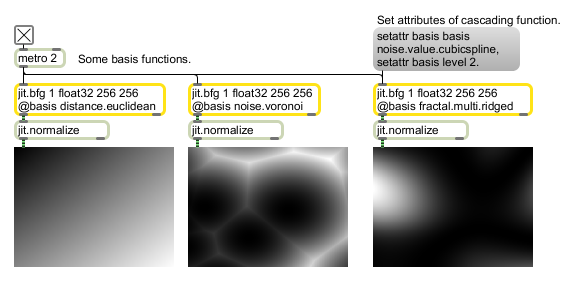# jit.bfg

Evaluates a procedural basis function graph

## Description

The jit.bfg object evaluates and exposes a library of procedural basis functions. Each of these basis functions can be evaluated in any number of dimensions, across any coordinate, without any need of referencing existing calculations. In addition, since they all share a common interface, basis functions can be combined together and evaluated in a function graph by cross-referencing several jit.bfg objects.
There are several categories of functions, each of which are characterized by a different intended use. These categories include fractal, noise, filter, transfer, and distance operations. Functions contained in these folders can be passed by name to jit.bfg either fully qualified (category.classname) or relaxed (classname).
By default, jit.bfg will generate spatial coordinates across a grid, however, if a jit.matrix is attached to the left-most inlet, the matrix's planar values will instead be used (planar values correspond to dimensions for the coordinates e.g. RGB == XYZ). After creation, jit.bfg requires a basis to be specified before any evaluation will be performed.

## Matrix Operator

matrix inputs:1, matrix outputs:1
 Name IOProc Planelink Typelink Dimlink Plane Dim Type out n/a 1 1 1 1 1 char long float32 float64

## Messages

 setattr The word setattr, followed by the name of an attribute and value or list of values, forwards attribute messages on to internal objects (e.g. setattr basis abs 0 would set the basis function object's abs attribute to zero.

## Attributes

Name Type g/s Description
align float The fractional alignment for offsetting each plane. (default = 10)
autocenter int Flag for enabling or disabling automatically placing the origin at the center of the output matrix. (default = 0)
basis symbol The name of the basis function to use for the evaulation. (default = none) Supported basis functions are:
Distance Functions
chebychev (Absolute maximum difference between two points)
euclidean (True straight line distance in Euclidean space)
euclidean.squared (Squared Euclidean distance)
manhattan (Rectilinear distance measured along axes at right angles)
minkovsky (Exponentially controlled distance)
Filter Functions
box (Sums all samples in the filter area with equal weight)
gaussian (Weights samples in the filter area using a bell curve)
lanczossinc (Weights samples using a steep windowed sinc curve)
mitchell (Weights samples using a controllable cubic polynomial)
disk (Sums all samples inside the filter's radius with equal weight)
sinc (Weights samples using an un-windowed sinc curve)
catmullrom (Weights samples using a Catmull-Rom cubic polynomial)
bessel (Weights samples with a linear phase response)
triangle (Weights samples in the filter area using a pyramid)
Transfer Functions
step (Always 0 if value is less than threshold, otherwise always 1)
smoothstep (Step function with cubic smoothing at boundaries)
bias (Polynomial similar to gamma but remapped to unit interval)
cubic (Generic 3rd order polynomial with controllable coefficients)
saw (Periodic triangle pulse train)
quintic (Generic 5th order polynomial with controllable coefficients)
gain (S-Shaped polynomial evaluated inside unit interval)
pulse (Periodic step function)
smoothpulse (Periodic step function with cubic smoothing at boundaries)
sine (Periodic sinusoidal curve)
linear (Linear function across unit interval)
solarize (Scales given value if threshold is exceeded)
Noise Functions
cellnoise (Coherent blocky noise)
checker (Periodic checker squares)
value.cubicspline (Polynomial smoothed pseudo-random values)
value.convolution (Convolution filtered pseudo-random values))
sparse.convolution (Convolution filtered pseudo-random feature points)
gradient (Directionally weighted polynomially interpolated values)
simplex (Simplex weighted pseudo-random values)
voronoi (Distance weighted pseudo-random feature points)
distorted (Domain distorted combinational noise)
Fractal Functions
mono (Additive fractal with global simularity across scales)
multi (Multiplicative fractal with varying simularity across scales)
multi.hybrid (A hybrid additive and multiplicative fractal)
multi.hetero (Heterogenous multiplicative fractal)
multi.ridged (Multiplicative fractal with sharp ridges)
turbulence (Additive mono-fractal with sharp ridges)
classname symbol The name of the basis function class (eg cellnoise) (default = none)
offset float The dimensional offsets to use for generating the spatial grid coordinates (only valid when an input matrix is not attached). (default = 0)
origin float The dimensional origin to use for generating the spatial grid coordinates (only valid when an input matrix is not attached). (default = 0)
precision symbol Internal precision for to use for evaluation, independent of matrix datatype. (default = float32)
rotation float The rotation angles to use for generating the spatial grid coordinates (only valid when an input matrix is not attached). (default = 0)
scale float The dimensional scale factors to use for generating the spatial grid coordinates (only valid when an input matrix is not attached). (default = 0)
seed int The seed value to use for initializing the pseudo-random number generator for alignment. The same seed will result in the same output (default = -1138).
weight float The weight factors for scaling the output values. (default = 1)

## Examples